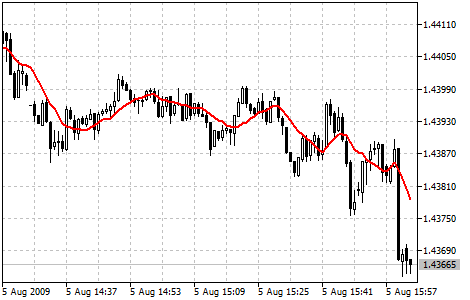# 移动均线

• 简单移动平均线 (SMA)
• 指数移动平均线 (EMA)
• 平滑移动平均线 (SMMA)
• 线形权数移动平均线 (LWMA)## 计算

### 简单移动平均线 (SMA) #

SMA = SUM (CLOSE (i), N) / N

SUM ― N周期求和;
CLOSE (i) ― 收盘价;
N ― 计算中使用到的周期。

### 指数移动平均线 (EMA) #

EMA = (CLOSE (i) * P) + (EMA (i - 1) * (1 - P))

CLOSE (i) ― 当前收盘价;
EMA (i - 1) ― 前一周期移动平均线值;
P ― 使用价格数值的百分比。

### 平滑移动平均线 (SMMA) #

SUM1 = SUM (CLOSE (i), N)

SMMA1 = SUM1 / N

SMMA (i) = (SMMA1*(N-1) + CLOSE (i)) / N

PREVSUM = SMMA (i - 1) * N

SMMA (i) = (PREVSUM - SMMA (i - 1) + CLOSE (i)) / N

SUM ― sum;
SUM1 ― total sum of closing prices for N periods; it is counted from the previous bar;
PREVSUM ― smoothed sum of the previous bar;
SMMA (i-1) ― smoothed moving average of the previous bar;
SMMA (i) ― smoothed moving average of the current bar (except for the first one);
CLOSE (i) ― current close price;
N ― smoothing period.

SMMA (i) = (SMMA (i - 1) * (N - 1) + CLOSE (i)) / N

### 线形权数移动平均线 (LWMA) #

LWMA = SUM (CLOSE (i) * i, N) / SUM (i, N)

SUM ― N周期求和;
CLOSE(i) ― 收盘价;
SUM (i, N) ― 权数系数总和;
N ― 平滑周期。